# Test: MCQs (One or More Correct Option): Modern Physics | JEE Advanced

## 38 Questions MCQ Test Class 12 Physics 35 Years JEE Mains &Advance Past year Paper | Test: MCQs (One or More Correct Option): Modern Physics | JEE Advanced

Description
Attempt Test: MCQs (One or More Correct Option): Modern Physics | JEE Advanced | 38 questions in 80 minutes | Mock test for JEE preparation | Free important questions MCQ to study Class 12 Physics 35 Years JEE Mains &Advance Past year Paper for JEE Exam | Download free PDF with solutions
*Multiple options can be correct
QUESTION: 1

### The shortest wavelength of X-rays emitted from an X-ray tube depends on

Solution:

Note : Shortest wavelength means highest frequency.
This means highest energy.
The energy of X-rays depends on the accelerating voltage provided in the X-ray tube.
Also, accordin g to Moseley’s law √n= a(Z –b) .
Thus the frequency also depends on the atomic number.

*Multiple options can be correct
QUESTION: 2

### The threshold wavelength for photoelectric emission from a material is 5200 Å. Photoelectrons will be emitted when this material is illuminated with monochromatic radiation from a

Solution:

The threshold wavelength is 5200Å. For ejection of electrons, the wavelength of the light should be less than 5200 Å, so that frequency increases and hence the energy of incident photon increases. U.V light has less wavelength than 5200 Å.

*Multiple options can be correct
QUESTION: 3

### From the following equations pick out the possible nuclear fusion reactions

Solution:

Nuclear fusion occurs when two or more lighter nuclei combine to form a heavier nucleus with release of a huge amount of energy.

*Multiple options can be correct
QUESTION: 4

In Bohr ’s model of the hydrogen atom

Solution:

We know, rn ∝ n2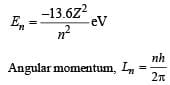| P.E. | = 2 × | K.E. |

*Multiple options can be correct
QUESTION: 5

Select the correct statement from the following

Solution:

A diode can be used as a rectifier.

*Multiple options can be correct
QUESTION: 6

For a given plate voltage, the plate current in a triode valve is maximum when the potential of

Solution:

is the correct option. The electrons emitted by emitter are collected to the maximum by the plate in this case.

*Multiple options can be correct
QUESTION: 7

The X-ray beam coming from an X-ray tube will be

Solution:

is the correct option.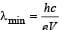*Multiple options can be correct
QUESTION: 8

The mass number of a nucleus is

Solution:

In the case of hydrogen, atomic number = mass number In the other atoms, atomic number < mass number.

*Multiple options can be correct
QUESTION: 9

Four physical quantities are listed in Column I. Their values are listed in Column II in a random order: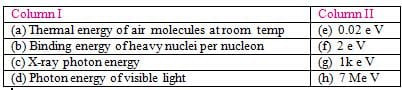The correct matching of Columns I and II is given by

Solution:
*Multiple options can be correct
QUESTION: 10

Photoelectric effect suppor ts quantum nature of light because

Solution:
*Multiple options can be correct
QUESTION: 11

During a negative beta decay

Solution:
*Multiple options can be correct
QUESTION: 12

During a nuclear fusion reaction

Solution:
*Multiple options can be correct
QUESTION: 13

The potential difference applied to an X-ray tube is increased. As a result, in the emitted radiation

Solution:
*Multiple options can be correct
QUESTION: 14

A freshly prepared radioactive source of half life 2 hr emits radiation of intensity which  is 64 times the permissibe safe level. The minimum time after which it would be possible to work safely with this source is

Solution:

Note:  The intensity of radiation emitted is proportional to the rate of decay which in turn is proportional to number of atoms left (radioactive).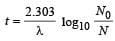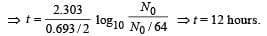*Multiple options can be correct
QUESTION: 15

The impurity atoms with which pure silicon should be doped to make a p-type semiconductor are those of

Solution:

Boron and Aluminium are trivalent impurities.

*Multiple options can be correct
QUESTION: 16

Two identical p-n junctions may be connected in series with a battery in three ways, fig. The potential drops across the two p – n junctions are equal in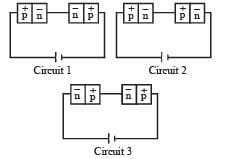Solution:

Since the p-n junction arrangement are in series, therefore the potential drop across a p-n junction will be proportional to their resistances. When the resistances will be equal, the potential drops will be equal. In circuit I, the two p-n junctions are attached such that one is forward biased (low resistance) and other is reverse biased (high resistance). Whereas in the other two circuits both are either forward biased or reversed biased.

*Multiple options can be correct
QUESTION: 17

The decay constant of a radioactive sample is λ . The halflife and mean-life of the sample are respectively given by

Solution: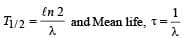*Multiple options can be correct
QUESTION: 18

When a monochromatic point source of light is at a distance of 0.2 m from a photoelectric cell, the cut off voltage and the saturation current are respectively 0.6 V and 18.0 mA. If the same source is placed 0.6 m away from the photoelectric cell, then

Solution:

Since the stopping potential depends on the frequency and not on the intensity and the source is same, the stopping potential remains unaffected. The saturation current depends on the intensity of incident light on the cathode of the photocell which in turn depends on the distance of the source from cathode. The intensity (I) of light is inversely proportional to the square of the distance between the light source and photocell.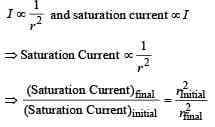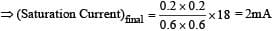*Multiple options can be correct
QUESTION: 19

In an n-p-n transistor circuit, the collector current is 10 mA. If 90% of the electrons emitted reach the collector,

Solution:

Ic = 10 mA

90% of electrons emitted pr produce a collector current of 10 mA. The base current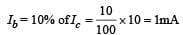Now, Ie = Ib + Ic = 1 + 10 = 11mA

*Multiple options can be correct
QUESTION: 20

A star initially has 1040 deuterons. It produces energy via the processes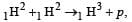and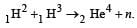If the average power radiated by the star is 1016 W, the deuteron supply of the star is exhausted in a time of the order of

The masses of the nuclei are as follows :

M (H2) = 2.014 amu;
M (p) = 1.007 amu; M(n) = 1.008 amu; M (He4) = 4.001amu.

Solution:

1H2 + 1H21H3 + p
1H2 + 1H32He4 + n
Net Reaction 31H22He4 + p + n
Δm = 3 (2.014) – [4.001 + 1.007 + 1.008] = 0.026
3 deuterons release 3.87 × 10–12 J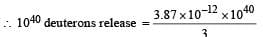= 1.29 × 1028J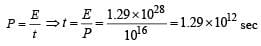*Multiple options can be correct
QUESTION: 21

When photons of energy 4.25 eV strike the surface of metal A, the ejected photoelectrons have maximum kinetic energy, TA eV and de Broglie wavelength λA. The maximum kinetic energy of photoelectrons liberated from another metal B by photons of energy 4.70 eV is TB = (TA- 1.50) eV. If the de Broglie wavelength of these photoelectrons is λB = 2λA, then

Solution:

For metal A

4.25 = WA + TA           ...(i)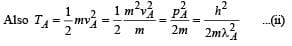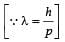For metal B

4.7 = (TA – 1.5) + WB ...(iii)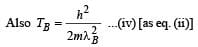Dividing equation (iv) by (ii),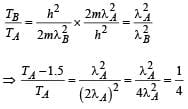[∵ λ B = 2λA given]

⇒ 4TA – 6 =TA ⇒ TA = 2 eV
From (i), WA = 2.25 eV
From (iii), WB = 4.2 eV
Also TB = TA – 1.5 ⇒ TB = 0.5eV

*Multiple options can be correct
QUESTION: 22

Which of the following statement(s) is (are) correct?

Solution:
*Multiple options can be correct
QUESTION: 23

Holes are charge carriers in

Solution:

Holes are electron vacancies which participate in electrical conductivity. These are produced in semiconductors.

*Multiple options can be correct
QUESTION: 24

A transistor is used in the common emitter mode as an amplifier. Then

Solution:

The circuit for a p-n-p transistor used in the common emitter mode as an amplifier is shown in figure. The base emitter junction is forward-biased and the input signal is connected in series with the voltage applied to bias the base emitter junction.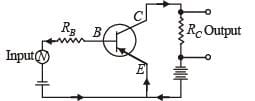*Multiple options can be correct
QUESTION: 25

Let mp be the mass of a proton, mn the mass of a neutron, M1 the mass of a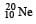nucleus and M2 the mass  of a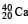nucleus. Then

Solution:

KEY CONCEPT : Due to mass defect (which is finally responsible for the binding energy of the nucleus), mass of a nucleus is always less than the sum of masses of its constituent particles.is made up of 10 protons plus 10 neutrons.
Therefore, mass ofnucleus
M1 < 10 (mp + mn)
Note :
Heavier the nucleus, more is the mass defect.
20 (mn + mp) – M2 > 10 (mp + mn) – M1
Thus, 10 (mn + mp) > M2 – M1
or M2 < M1 + 10 (mp + mn)
Now, since M1 < 10 (mp + mn)
∴ M2 < 2M1

*Multiple options can be correct
QUESTION: 26

The electron in a hydrogen atom makes a transition n→ n2 where n1 and n2 are the principal quantum numbers of the two states. Assume the Bohr model to be valid. The time period of the electron in the initial state is eight times that in the final state. The possible values of n1 and n2 are

Solution:

The time period of the electron in a Bohr orbit is given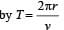Since for the nth Bohr orbit, mvr = n (h/2π), the time period becomes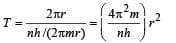Since the radius of the orbit r depends on n, we replace r. Bohr radius of a hydrogen atom is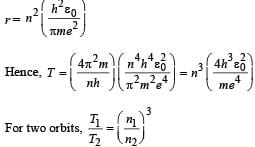It is given that T1 / T2 = 8, hence, n1/n2 = 2.

*Multiple options can be correct
QUESTION: 27

The half-life of 131I is 8 days. Given a sample of 131I at time t = 0, we can assert that

Solution:

The result follows from the formula based on laws of radioactive decay N = N0e–λt The nucleus start decaying after time t = 0

*Multiple options can be correct
QUESTION: 28

In a p-n junction diode not connected to any circuit,

Solution:

At junction a potential barrier/depletion layer is formed as shown, with n-side at higher potential and p-side at lower potential. Therefore, there is an electric field at the junction directed from the n-side to p-side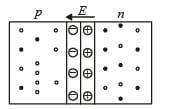*Multiple options can be correct
QUESTION: 29

X-rays are produced in an X-ray tube operating at a given accelerating voltage. The wavelength of the continuous X-rays has values from

Solution:

The continuous X-ray spectrum is shown in figure.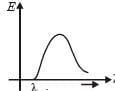All wavelengths > λmin are found.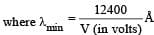Here V is the applied voltage.

*Multiple options can be correct
QUESTION: 30

The work function of a substance is 4.0 eV. The longest wavelength of light that can cause photoelectron emission from this substance is approximately

Solution: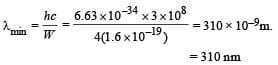*Multiple options can be correct
QUESTION: 31

The half-life period of a radioactive element X is same as the mean-life time of another radioactive element Y. Initially both of them have the same number of atoms. Then

Solution: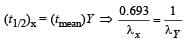∴ λx = 0.693 λY
λx < λY. Now, rate of decay = λN
Initially, number of atoms (N) of both are equal but since λY < λx, therefore Y will decay at a faster rate than x.

*Multiple options can be correct
QUESTION: 32

The graph between the stopping potential (V0) and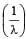is shown in the figure. φ1 , φ2 and φ3 are work functions, which of the following is/are correct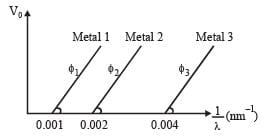Solution: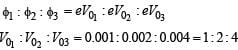Therefore option (a) is correct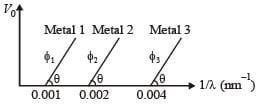By Einstein’s photoelectric equation,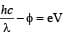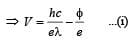Comparing equation (i) by y = mx + c, we get the slope of the line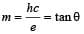⇒ Option (c) is correct.
From the graph it is clear that,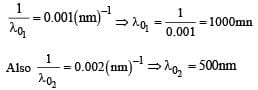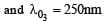Note : Violet colour light will have wavelength less than 400 nm.
Therefore, this light will be unable to show photoelectric effect on plate 3 ⇒Þ Option (d) is wrong.

*Multiple options can be correct
QUESTION: 33

Assume that the nuclear binding energy per nucleon (B/A) versus mass number (A) is as shown in the figure. Use this plot to choose the correct choice(s) given below.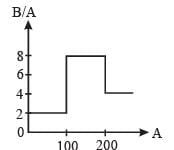Solution:

Note : When binding energy per nucleon increases for a nuclear process, energy is released. 
When two nuclei of mass numbers between 51 to 100 fuse, the mass number of the resulting nuclei will come out to be between 100 to 200. The graph shows that in this process the binding energy per nucleon increases and therefore energy is released. 
When nucleus of mass number 200 to 260 breaks; it will produce nuclei of mass numbers lying between 100 to 200 if we assume that the two daughter nuclei are of nearly same mass. This in fact happens practically that when a heavy nucleus splits into two parts during nuclear fission, two moderate size nuclei are formed in general. The graph shows that in this process also the binding energy per nucleon increases. Therefore energy is released.

*Multiple options can be correct
QUESTION: 34

The radius of the orbit of an electron in a Hydrogen-like atom is 4.5 a0, where a0 is the Bohr radius. Its orbital angular momentum is 3h/2π It is given that h is Planck constant and R is Rydberg constant. The possible wavelength(s), when the atom de-excites, is (are)

Solution:

Angular momentum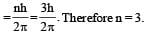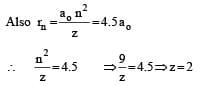we know that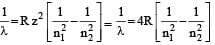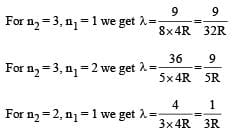(a), (c) are correct options

*Multiple options can be correct
QUESTION: 35

For photo-electric effect with incident photon wavelength λ, the stopping potential is V0. Identify the correct variation(s) of V0 with λ and 1/λ.

Solution: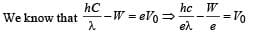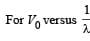we should get a straight line with negativeslope and positive intercept.
For V0 vesus λ, we will get a hyperbola. As λ decreases V0 increases. (a) and (c) are the correct options

*Multiple options can be correct
QUESTION: 36

A fission reaction is given by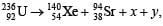where x and y are two particles. Considering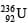to be at rest, the kinetic energies of the products are denoted by KXe, KSr, Kx(2 MeV) and Ky(2 MeV), respectively. Let the binding energies per nucleon of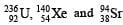be 7.5 MeV, 8.5 MeV and 8.5 MeV, respectively. Considering different conservation laws, the correct option(s) is(are)

Solution: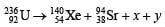The number of proton in reactants is equal to the products (leaving x and y) and mass number of product (leaving x and y) is two less than reactants
∴ x = p, y = e is ruled out [B] is incorrect and x = p, y = n is ruled out [C] is incorrect

Total energy loss = (236 × 7.5) – [140 × 8.5 + 94 × 8.5] = 219 MeV
The energies of kx and ky together is 4MeV The energy remain is distributed by Sr and Xe which is equal to 219 – 4 = 215 MeV
∴ A is the correct option Also momentum is conserved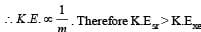The energies of kx and ky together is 4MeV The energy remain is distributed by Sr and Xe which is equal to 219 – 4 = 215 MeV

∴ A is the correct option Also momentum is conserved .
Therefore K.Esr > K.Exe

*Multiple options can be correct
QUESTION: 37

Highly excited states for hydrogen–like atoms (also called Rydberg states) with nuclear charge Ze are defined by their principal quantum number n, where n>>1. Which of the following statement(s) is(are) true?

Solution: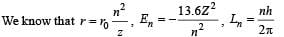Relative change in the radii of two consecutive orbitals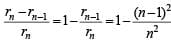does not depend on Z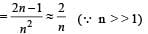Relative change in the energy of two consecutive orbitals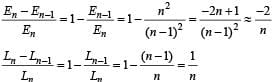*Multiple options can be correct
QUESTION: 38

Light of wavelength λph falls on a cathode plate inside a vacuum tube as shown in the figure. The work function of the cathode surface is φ and the anode is a wire mesh of conducting material kept at a distance d from the cathode. A potential difference V is maintained between the electrodes.
If the minimum de Broglie wavelength of the electrons passing through the anode is λe, which of the following statement(s) is (are) true?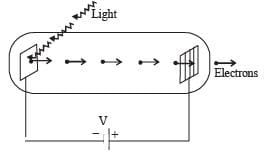Solution:

The wavelength of emitted photoelectron as per de Broglie is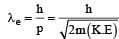When φ increases, K.E. decreases and therefore λe increases
When λph increases, Nph decreases , K.E decreases and therefore λe increases. le is independent of the distance d.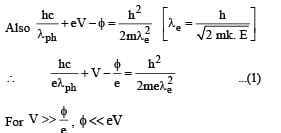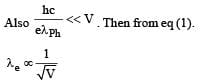Therefore if V is made our times, λe is approximately half.Use Code STAYHOME200 and get INR 200 additional OFF Use Coupon Code